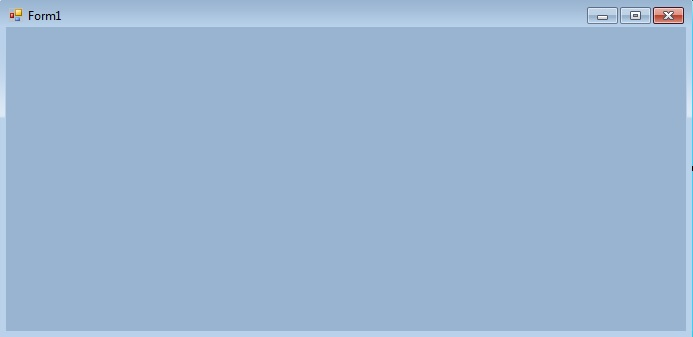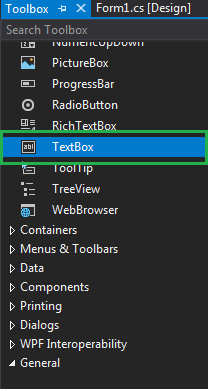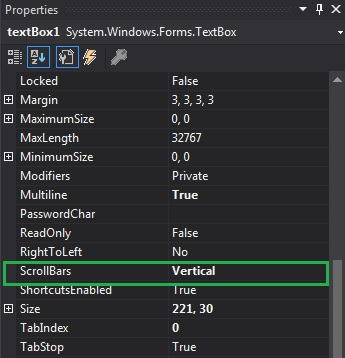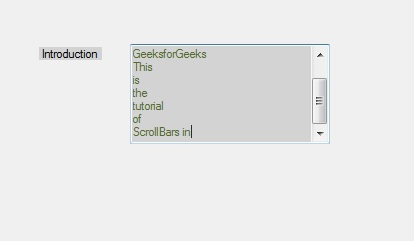# How to set Scrollbar in TextBox in C#?

• Last Updated : 29 Nov, 2019

In Windows forms, TextBox plays an important role. With the help of TextBox, the user can enter data in the application, it can be of a single line or of multiple lines. In TextBox, you are allowed to set scrollbars when you are working with multiline TextBox with the help of ScrollBars property of the TextBox. The default value of this property is in Windows form, you can set this property in two different ways:

1. Design-Time: It is the simplest way to set the ScrollBars property of the TextBox as shown in the following steps:

• Step 1: Create a windows form.
Visual Studio -> File -> New -> Project -> WindowsFormApp• Step 2: Drag the TextBox control from the ToolBox and Drop it on the windows form. You can place TextBox anywhere on the windows form according to your need. As shown in the below image:• Step 3: After drag and drop you will go to the properties of the TextBox control to set the ScrollBars property of the TextBox.Output:Note: Please remember the horizontal scroll bars will not be shown if the WordWrap property is set to true, regardless of the value of the ScrollBars property.

2. Run-Time: It is a little bit trickier than the above method. In this method, you can set the ScrollBars property of the TextBox programmatically with the help of given syntax:

`public System.Windows.Forms.ScrollBars ScrollBars { get; set; }`

Here, the ScrollBars represent the ScrollBars enumeration values that indicate whether a multiline TextBox control appears with no scroll bars, a horizontal scroll bar, a vertical scroll bar, or both. And it will throw an InvalidEnumArgumentException if the value that is assigned to the property is not within the range of valid values for the enumeration. Following steps are used to set the ScrollBars property of the TextBox:

• Step 1 : Create a textbox using the TextBox() constructor provided by the TextBox class.
```// Creating textbox
TextBox Mytextbox = new TextBox();
```
• Step 2 : After creating TextBox, set the ScrollBars property of the TextBox provided by the TextBox class.
```// Set ScrollBars property
Mytextbox.ScrollBars = ScrollBars.Vertical;
```
• Step 3 : And last add this textbox control to from using Add() method.
```// Add this textbox to form
```

Example:

 `using` `System;``using` `System.Collections.Generic;``using` `System.ComponentModel;``using` `System.Data;``using` `System.Drawing;``using` `System.Linq;``using` `System.Text;``using` `System.Threading.Tasks;``using` `System.Windows.Forms;`` ` `namespace` `my {`` ` `public` `partial` `class` `Form1 : Form {`` ` `    ``public` `Form1()``    ``{``        ``InitializeComponent();``    ``}`` ` `    ``private` `void` `Form1_Load(``object` `sender, EventArgs e)``    ``{``        ``// Creating and setting the properties of Lable1``        ``Label Mylablel = ``new` `Label();``        ``Mylablel.Location = ``new` `Point(96, 54);``        ``Mylablel.Text = ``"Introduction"``;``        ``Mylablel.AutoSize = ``true``;``        ``Mylablel.BackColor = Color.LightGray;`` ` `        ``// Add this label to form``        ``this``.Controls.Add(Mylablel);`` ` `        ``// Creating and setting the properties of TextBox1``        ``TextBox Mytextbox = ``new` `TextBox();``        ``Mytextbox.Location = ``new` `Point(187, 51);``        ``Mytextbox.BackColor = Color.LightGray;``        ``Mytextbox.ForeColor = Color.DarkOliveGreen;``        ``Mytextbox.Height = 100;``        ``Mytextbox.Width = 200;``        ``Mytextbox.Name = ``"text_box1"``;``        ``Mytextbox.Multiline = ``true``;``        ``Mytextbox.ScrollBars = ScrollBars.Vertical;`` ` `        ``// Add this textbox to form``        ``this``.Controls.Add(Mytextbox);``    ``}``}``}`

Output:My Personal Notes arrow_drop_up